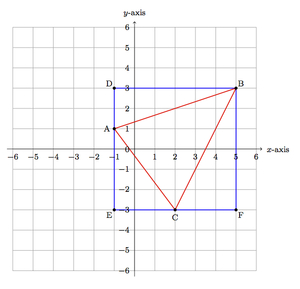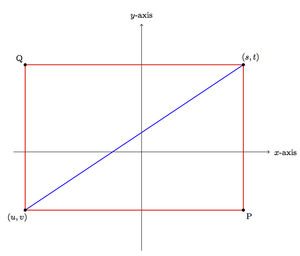# Finding the distance between points

Alignments to Content Standards: 8.G.B.8

1. Plot the points (5,3), (-1,1), and (2,-3) in the coordinate plane and find the lengths of the three segments connecting the points.
2. Find the distance between (5,9) and (-4,2) without plotting the points.
3. If $(u,v)$ and $(s,t)$ are two distinct points in the plane, what is the distance between them? Explain how you know.

## IM Commentary

The goal of this task is to establish the distance formula between two points in the plane and its relationship with the Pythagorean Theorem. Students should already be familiar with applying the Pythagorean in concrete situations such as https://www.illustrativemathematics.org/illustrations/1417 . The teacher will want to make sure that students explain their calculations in parts (a) and (b). For each segment length calculation in (a), this will mean drawing a right triangle which has the given segment as one of its sides. The simplest and most uniform way to do this is to use coordinate grid lines which are perpendicular by construction. For part (b), students need to analyze carefully their work for part (a) in order to relate the side lengths of the right triangles to the coordinates of the points. In part (c), students can make an abstract drawing similar to part (a) or they can build on the calculations in part (b). If students draw a picture for part (c), as is done in the solution, the teacher should show samples where the points lie in different quadrants or on one or both of the axes. This will help students see that the location of the points does not influence the calculations: only their relative position (found by taking differences of coordinates) is important. Part (d) has been added to encourage students to put all of their work together and verify that it is consistent. They can test the formula they have found in part (c) with any of the examples from (a) and (b).

One issue which will likely come up in this task is the order of the points and the signs of their differences. Looking at (-1,1) and (2,3) as an example, students may find  3 and 2 for the change in $x$ and $y$ coordinates or they may find -3 and -2. Either is viable, since when we apply the Pythagorean theorem we will square the quantities and $3^2 + 2^2 = (-3)^2 + (-2)^2$. These calculations build on and reinforce student understanding of distance and absolute value developed in 6.NS.7 and 7.NS.2.

## Solution

1. The three points and the segments joining them are plotted and labeled below:To calculate the distance between two of the points, $A$ and $B$ for example, we can use the Pythagorean Theorem provided we can find a right triangle which has $\overline{AB}$ as one side. The vertical and horizontal grid lines are perpendicular to one another so we can make a right angle by choosing one vertical grid line segment and one horizontal grid line segment as the legs of our triangle. This is also pictured above as $\triangle ADB$ is a right triangle with right angle $D$. We can apply the Pythagorean theorem to $\triangle ADB$ to find $$|AB|^2 = |AD|^2 + |BD|^2.$$We know $|AD| = 2$ units and $|BD| = 6$ units by counting squares on the coordinate grid. So this means $|AD| = \sqrt{40}$ units. Applying the same technique to $\triangle AEC$ with right angle $E$ we find $|AC| = \sqrt{25} = 5$ units. Using $\triangle CFB$ we find that $|CB| = \sqrt{45}$ units.

2. Building on the work in part (a), we can look for a pattern in the calculations. In each case we take a square root and we are taking the square root of the square of the horizontal change in the point coordinates added to the square of the vertical change in the point coordinates. Applying this idea to (5,9) and (-4,2), the horizontal change in these coordinates is $5 -(-4) = 9$ while the vertical change is $9-2=7$. The squares of these numbers are $9^2 = 81$ and $7^2 = 49$. Then the square root of the sum of these squares is $\sqrt{81+49} = \sqrt{130}$. So the segment joining $(5,9)$ to $(-4,2)$ has length $\sqrt{130}$ units.
3. Looking at part (b), we need to find the horizontal and vertical distance covered to go from $(u,v)$ to $(s,t)$. Moving from $u$ to $s$ requires a horizontal displacement of $s-u$ units while going from $v$ to $t$ is a vertical displacement of $t-v$ units. Applying the pattern in part (b), the distance from $(u,v)$ to $(s,t)$ is $\sqrt{(s-u)^2 + (t-v)^2}$.

We can verify this formula and pattern by drawing a representative picture as in part (a), though we have not labeled the numbers on the axes because we do not know the exact coordinates of the points:There are many other possible pictures as $(u,v)$ or $(s,t)$ could lie on one of the coordinate axes or could be in different quadrants. The Pythagorean relationship, however, holds regardless of where we translate the image.

In order to find the distance from $(u,v)$ to $(s,t)$ we can make these points two vertices of a rectangle, with horizontal and vertical sides, as drawn above. We let $A = (s,t)$ and $B = (u,v)$ in the following calculations. Since side $\overline{BQ}$ is vertical, this means that the $x$-coordinate of $Q$ is the same as that of $B=(u,v)$, namely $u$. Similarly, the $y$-coordinate of $Q$  is the same as the $y$-coordinate of $A = (s,t)$, namely $t$. So $Q = (u,t)$. Leg $\overline{BQ}$ has length $|t-v|$ while leg $QA$ has length $|s-u|$. Applying the Pythagorean theorem we find that the distance from $(u,v)$ to $(s,t)$ is $\sqrt{|s-u|^2 + |t-v|^2}$. This is the same as what we found above: the square of any number is non-negative so $|s-u|^2 = (s-u)^2$ and $|t-v|^2 = (t-v)^2$.

4. Here we apply the formula found in part (c) with $(u,v) = (5,3)$ and $(s,t) = (-1,1)$. We find that the distance between these points is $\sqrt{(-1-5)^2 + (1-3)^2} = \sqrt{40}$, agreeing with our calculations in part (a). The formula gives the same answers as we calculated above in all of the rest of the cases as well.